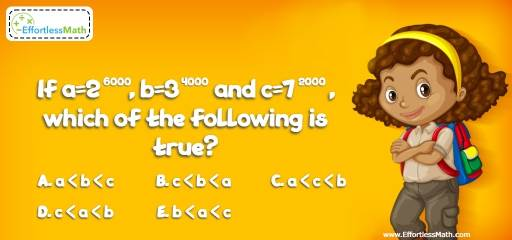# Number Properties Puzzle – Challenge 10

This is a great math challenge related to Number Properties for those who love critical thinking challenges. To solve this problem, you need to use your knowledge of exponents. Let's challenge your brain!## Challenge:

If $$a=2^{6000}, b=3^{4000}$$ and $$c=7^{2000}$$, which of the following is true?

A- $$a < b < c$$

B- $$c < b < a$$

C- $$a < c < b$$

D- $$c < a < b$$

E- $$b < a < c$$

### The Absolute Best Book to Challenge Your Smart Student!

$$a=2^{6000}, b=3^{4000}$$ and $$c=7^{2000}$$
Find the $$2000^{th}$$ rout of each number:
$$\sqrt{a}= \sqrt{2^{6000}} = 2^{\frac{6000}{2000}} = 2^3 = 8$$
$$\sqrt{b} =\sqrt{3^{4000}} = 3^{\frac{4000}{2000}} = 3^2 = 9$$
$$\sqrt{c} = \sqrt{7^{2000}} = 7^{\frac{2000}{2000}} = 7^1 = 7$$
Therefore: $$c < a < b$$

The Absolute Best Books to Ace Algebra

### What people say about "Number Properties Puzzle – Challenge 10 - Effortless Math: We Help Students Learn to LOVE Mathematics"?

No one replied yet.

X
45% OFF

Limited time only!

Save Over 45%

SAVE $40 It was$89.99 now it is \$49.99